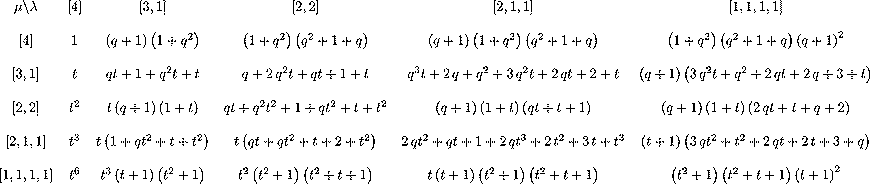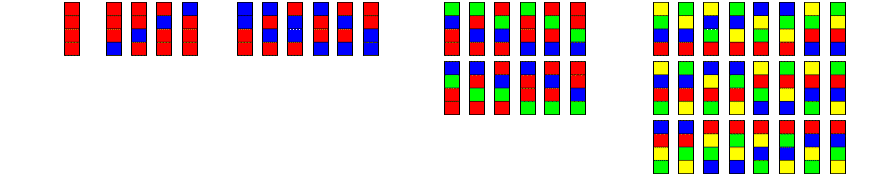HOME: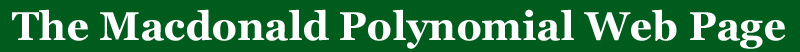BACK: More qt-analogs

# q,t-Binomial coefficients

The q,t-binomial coefficients are the coefficients of m_\lambda in the symmetric function Hµ(X;q,t) (or the wiggled version).  Below we show some tables of these polynomials.  The conjecture that they are polynomials with non-negative integer coefficients follows from the same result for the q,t-Kostka polynomials.  There is an interesting pair of relations between the Kostka coefficients and the binomial coefficients that extends to the q,t case.  Below are only the tables through n=4, TEX, postscript and PDF versions of these tables through n=5 are also available.

These coefficients were remarked to be q,t analogs of the binomial coefficients in the paper Affine Hecke algebras and raising operators for Macdonald polynomials by A. Kirillov and M. Noumi.  When lambda is a hook they were remarked to be a q,t analog of the falling factorial (but with the wiggled Kostka coefficients and an additional formula) in the article Identities and Positivity Conjectures for Some Remarkable Operators in the Theory of Symmetric Functions ( F. Bergeron, A. M. Garsia, M. Haiman and G. Tesler ).

 n=2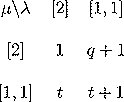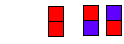n=3n=4